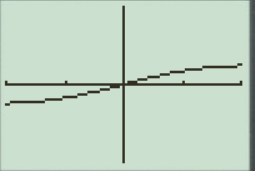# To graph : The quadratic equation y = x x 2 + 25 in a graphing window.### Precalculus: Mathematics for Calcu...

6th Edition
Stewart + 5 others
Publisher: Cengage Learning
ISBN: 9780840068071### Precalculus: Mathematics for Calcu...

6th Edition
Stewart + 5 others
Publisher: Cengage Learning
ISBN: 9780840068071

#### Solutions

Chapter 1.9, Problem 20E
To determine

## To graph: The quadratic equation y=xx2+25 in a graphing window.

Expert Solution

### Explanation of Solution

Given information:

Graph:

The graph of the quadratic equation y=xx2+25 can be sketched in the cartesian plane,

Consider the quadratic equation, y=xx2+25 .

Now put the values of x in the equation to find the values of y or to find the real roots of y with the help of x .

Rewrite the equation as numerator and denominator by x in RHS:

y=xx2+25y=1x+25x

Logic:

a+ka .

Minimum value:

a=kaa2=ka=k .

Max when a is ,

x+25xx=25xx2=25x=25

The value of x is 5 .

Now substitute the value of x=0 in equation y=xx2+25 .

y=xx2+25y=002+25y=0

Now put the value of x=1 in the equation, y=xx2+25 .

y=xx2+25y=112+25y=126

Substitute the value x=2 in the equation y=xx2+25 :

y=xx2+25y=222+25y=24+25y=229

Substitute the value x=3 in the equation y=xx2+25 :

y=xx2+25y=332+25y=39+25y=334

Substitute the value x=1 in the equation y=xx2+25 :

y=xx2+25y=112+25y=124

Substitute the value x=2 in the equation y=xx2+25 :

y=xx2+25y=222+25y=24+25y=229

Now the value of y is:

y=24+25y=229y=229

Substitute the value x=3 in the equation y=xx2+25 :

y=xx2+25y=332+25y=39+25y=334

Observed that when the value of x increases then the value of y decreases slightly.

When the value of x decreases then the value of y increases slightly.

Steps to plot the graph of the equation y=xx2+25 with the help of graphing utility are as follows:

Step 1: Press MODE key.

Step 2: Use the down arrow key to reach FUNC option.

Step 3: Press ENTER key.

Step 4: Press Y= key.

Step 5: Enter the function xx2+25 .

Step 6: Press WINDOW key. Change the settings to

Xmin=0.6Xmax=0.6Ymin=0.6Ymax=0.6

For better view of graph.

Step 8: Press GRAPH key.

The result obtained on the screen is provided below,Interpretation:

The equation of the function y=xx2+25 represents a wave graph.

The x intercepts are the points on xaxis where the graph of the equation touches xaxis .

At x -axis y is always zero.

Recall that the graphical approach to solve the equation simultaneously.

Therefore, in the equation, y=xx2+25 there is no x intercepts.

Therefore, the equation y=xx2+25 is symmetric about the y axis Initially the graph of the function y=xx2+25 increases when increase the value of x ,the graph is a parabola shaped graph.

### Have a homework question?

Subscribe to bartleby learn! Ask subject matter experts 30 homework questions each month. Plus, you’ll have access to millions of step-by-step textbook answers!